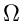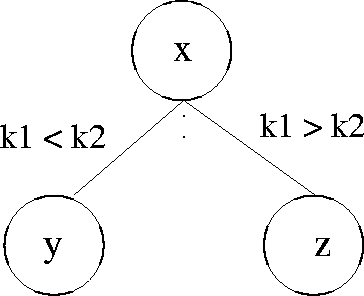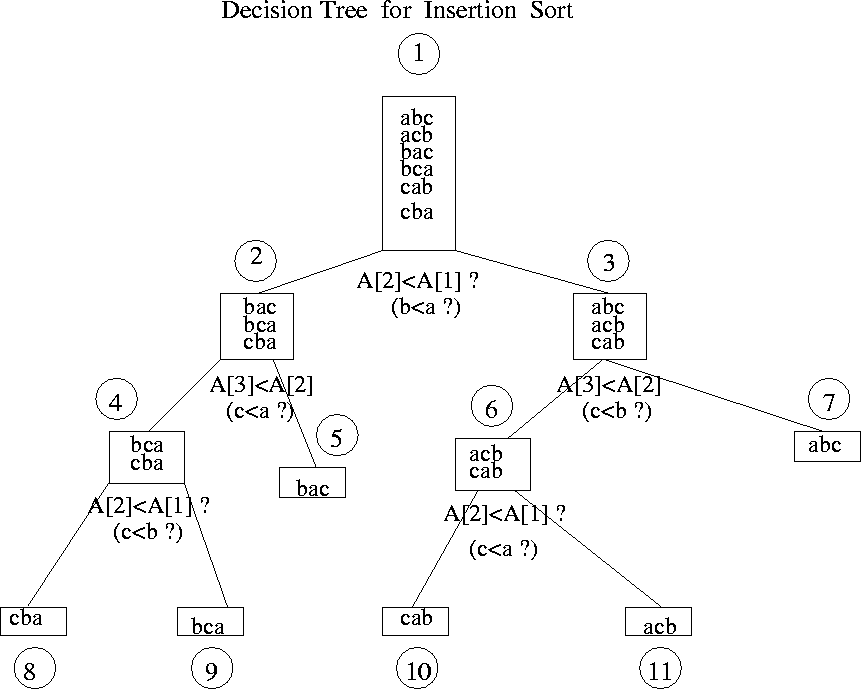Next:9.8.1 Result 1: Lower Bound on Worst Case ComplexityUp:9. Sorting MethodsPrevious:9.7.3 Algorithm 3

# 9.8 Lower Bound on Complexity for Sorting Methods

Result 1

The worst case complexity of any sorting algorithm that only uses key comparisons is(nlog n)

Result 2

The average case complexity of any sorting algorithm that only uses key comparisons is(nlog n)

The above results are proved using a Decision Tree which is a binary tree in which the nodes represent the status of the algorithm after making some comparisons.

Consider a node x in a decision tree and let y be its left child and zits right child. See Figure 9.4.Basically, y represents a state consisting of the information known at x plus the fact that the key k1 is less than key k2. For a decision tree for insertion sort on 3 elements, see Figure 9.5.Next:9.8.1 Result 1: Lower Bound on Worst Case ComplexityUp:9. Sorting MethodsPrevious:9.7.3 Algorithm 3
eEL,CSA_Dept,IISc,Bangalore